Скачать презентацию Lecture 10 ØRelationship Between Wavelength Frequency Energy and

26bee8c2709da6491aa59ff32bbdfab0.ppt

• Количество слайдов: 29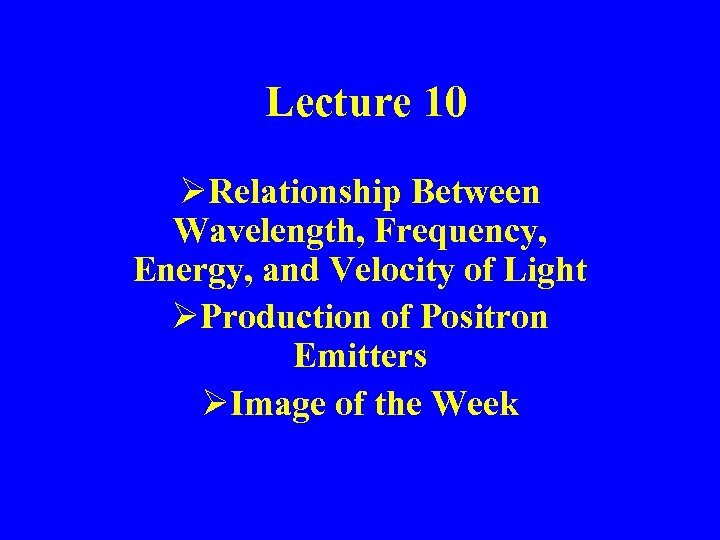Lecture 10 ØRelationship Between Wavelength, Frequency, Energy, and Velocity of Light ØProduction of Positron Emitters ØImage of the Week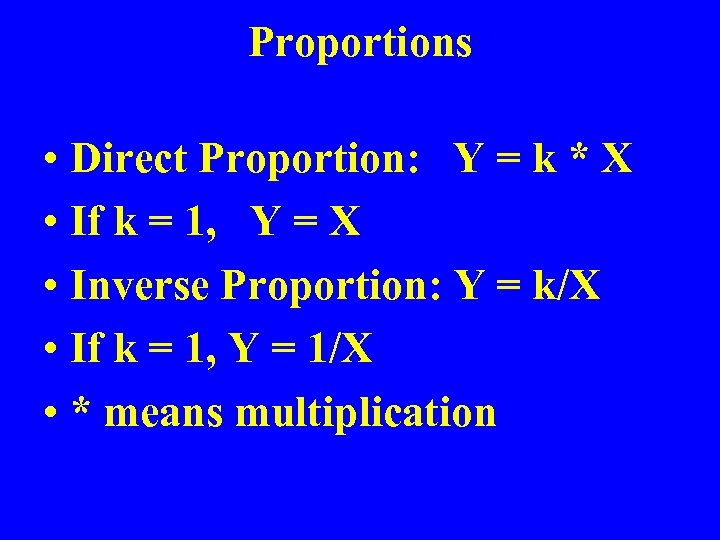Proportions • Direct Proportion: Y = k * X • If k = 1, Y = X • Inverse Proportion: Y = k/X • If k = 1, Y = 1/X • * means multiplication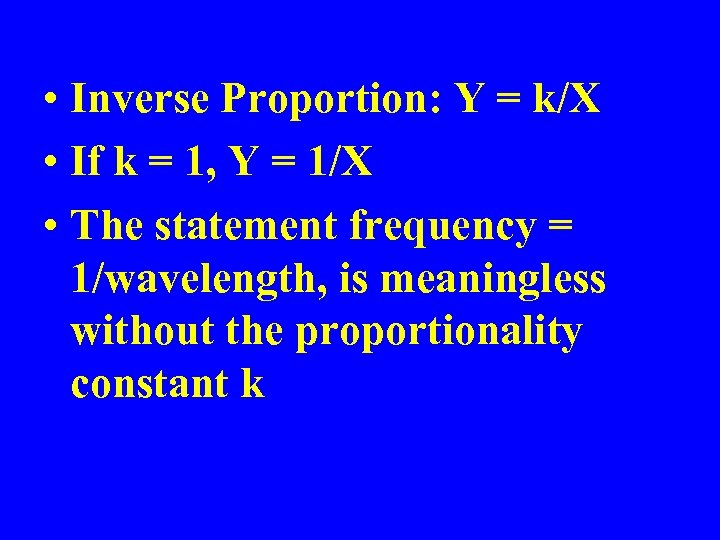• Inverse Proportion: Y = k/X • If k = 1, Y = 1/X • The statement frequency = 1/wavelength, is meaningless without the proportionality constant k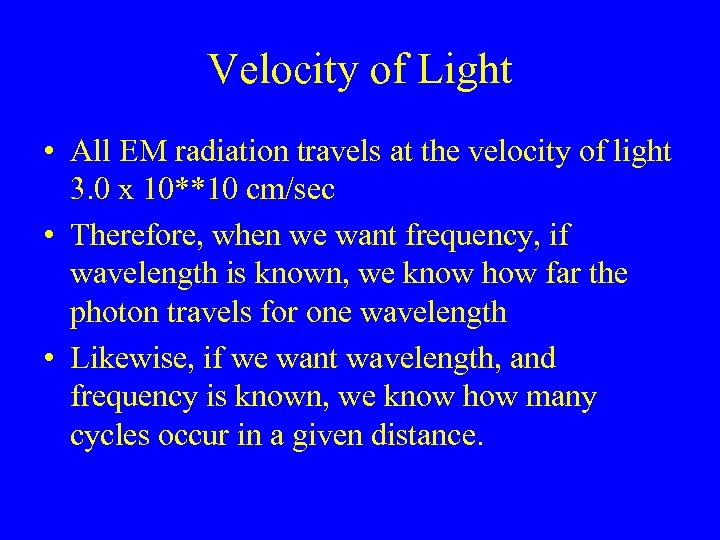Velocity of Light • All EM radiation travels at the velocity of light 3. 0 x 10**10 cm/sec • Therefore, when we want frequency, if wavelength is known, we know how far the photon travels for one wavelength • Likewise, if we want wavelength, and frequency is known, we know how many cycles occur in a given distance.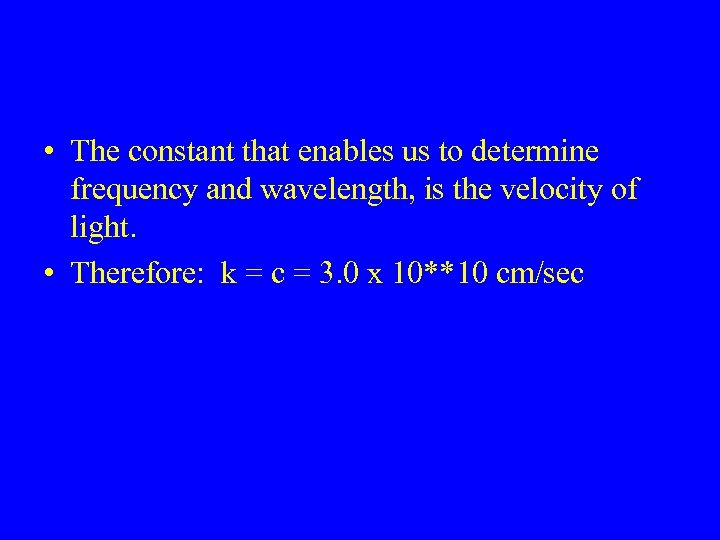• The constant that enables us to determine frequency and wavelength, is the velocity of light. • Therefore: k = c = 3. 0 x 10**10 cm/sec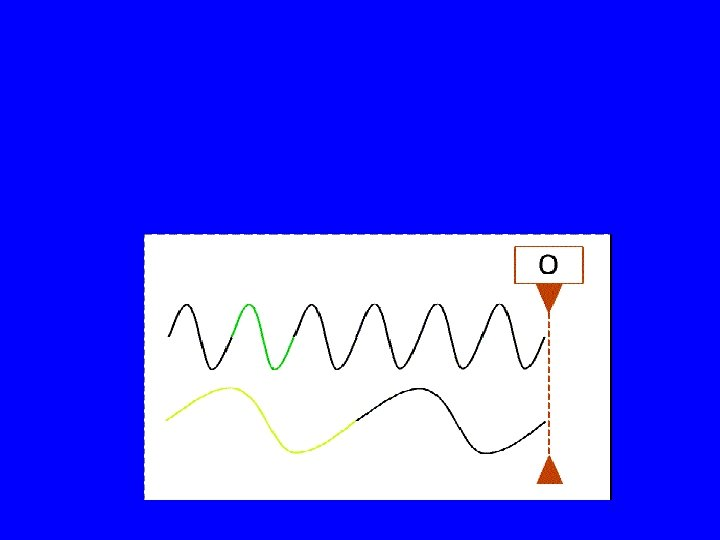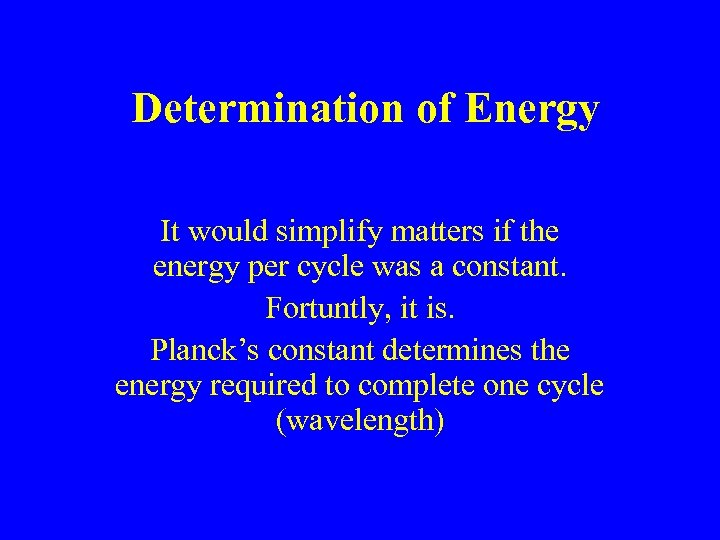Determination of Energy It would simplify matters if the energy per cycle was a constant. Fortuntly, it is. Planck’s constant determines the energy required to complete one cycle (wavelength)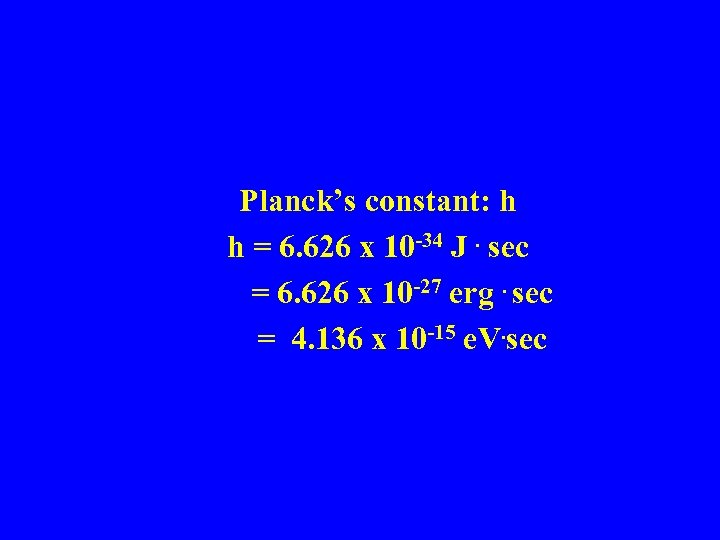Planck’s constant: h h = 6. 626 x 10 -34 J. sec = 6. 626 x 10 -27 erg. sec = 4. 136 x 10 -15 e. V. sec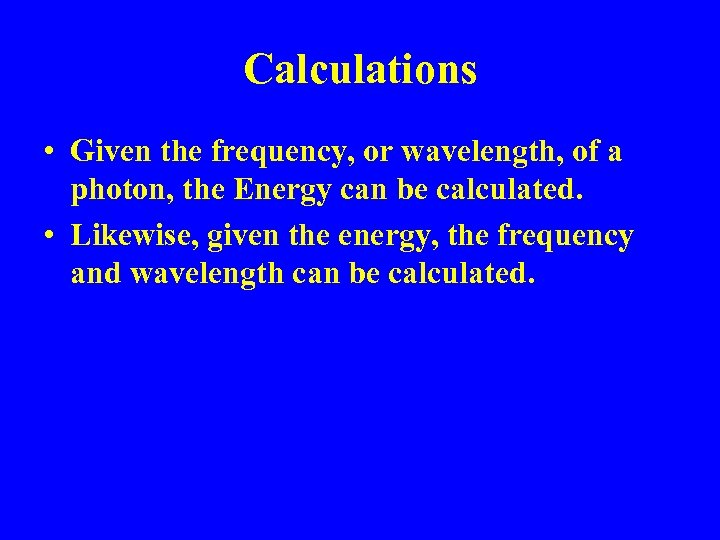Calculations • Given the frequency, or wavelength, of a photon, the Energy can be calculated. • Likewise, given the energy, the frequency and wavelength can be calculated.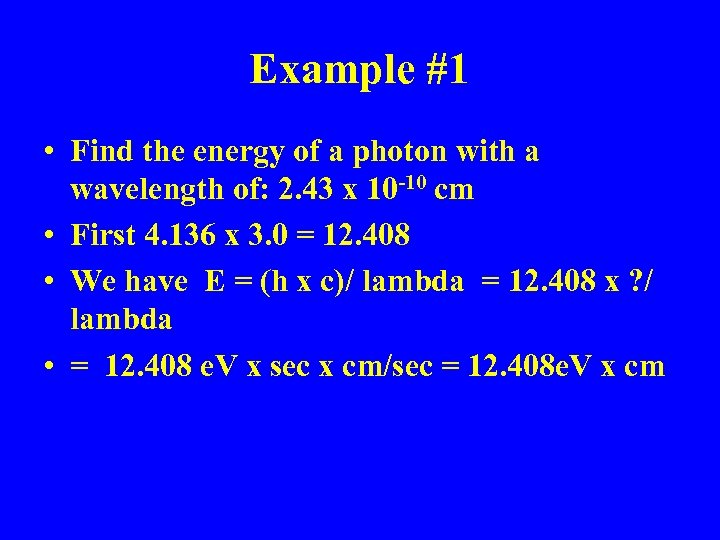Example #1 • Find the energy of a photon with a wavelength of: 2. 43 x 10 -10 cm • First 4. 136 x 3. 0 = 12. 408 • We have E = (h x c)/ lambda = 12. 408 x ? / lambda • = 12. 408 e. V x sec x cm/sec = 12. 408 e. V x cm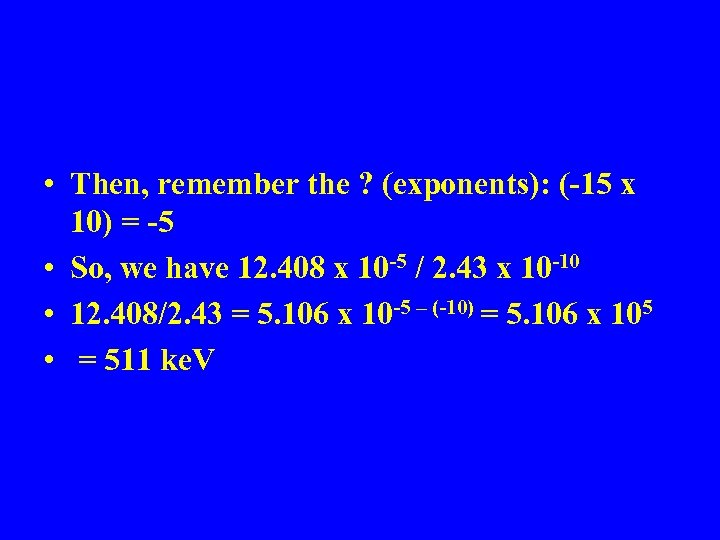• Then, remember the ? (exponents): (-15 x 10) = -5 • So, we have 12. 408 x 10 -5 / 2. 43 x 10 -10 • 12. 408/2. 43 = 5. 106 x 10 -5 – (-10) = 5. 106 x 105 • = 511 ke. V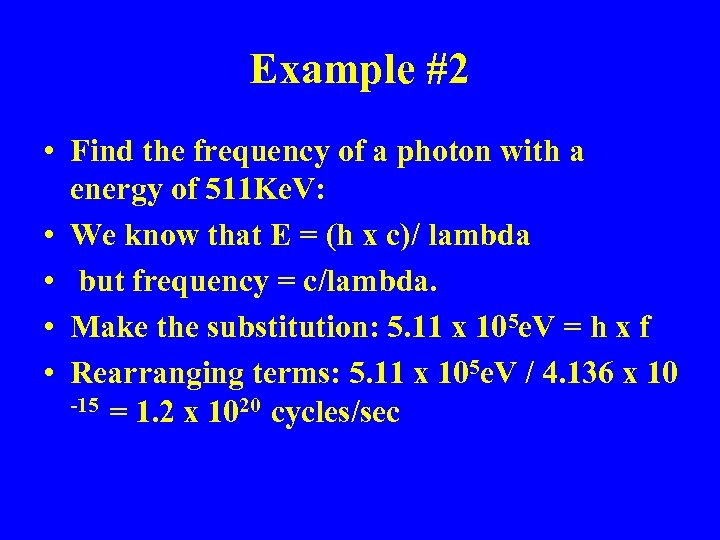Example #2 • Find the frequency of a photon with a energy of 511 Ke. V: • We know that E = (h x c)/ lambda • but frequency = c/lambda. • Make the substitution: 5. 11 x 105 e. V = h x f • Rearranging terms: 5. 11 x 105 e. V / 4. 136 x 10 -15 = 1. 2 x 1020 cycles/sec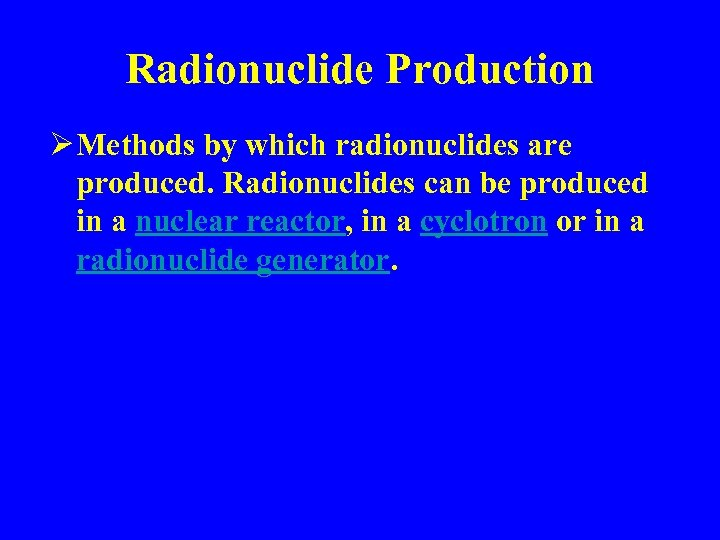Radionuclide Production Ø Methods by which radionuclides are produced. Radionuclides can be produced in a nuclear reactor, in a cyclotron or in a radionuclide generator.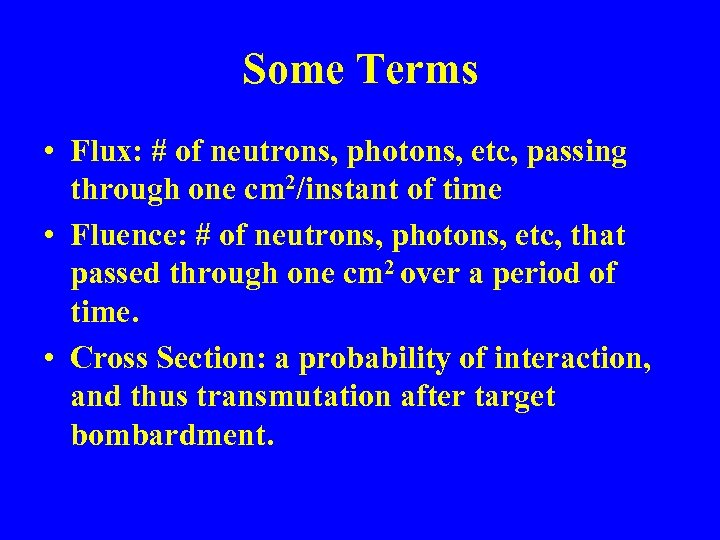Some Terms • Flux: # of neutrons, photons, etc, passing through one cm 2/instant of time • Fluence: # of neutrons, photons, etc, that passed through one cm 2 over a period of time. • Cross Section: a probability of interaction, and thus transmutation after target bombardment.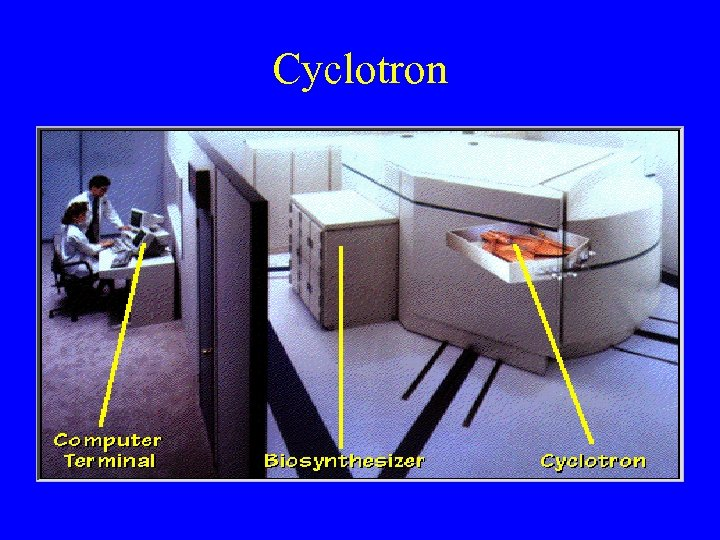Cyclotron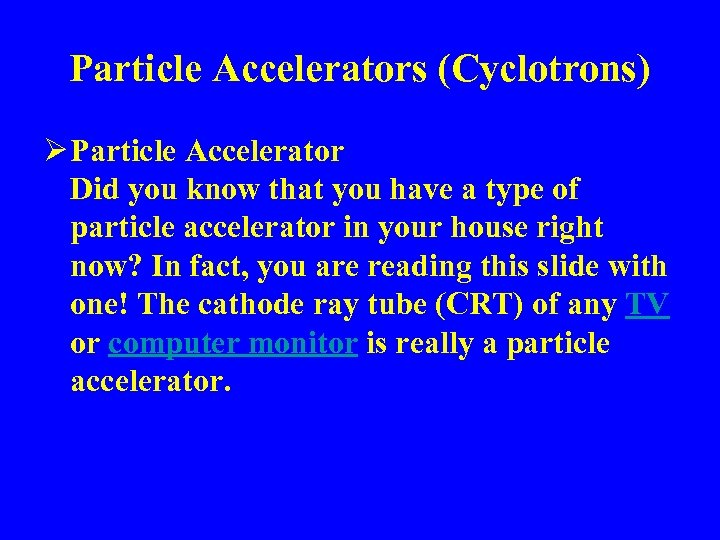Particle Accelerators (Cyclotrons) Ø Particle Accelerator Did you know that you have a type of particle accelerator in your house right now? In fact, you are reading this slide with one! The cathode ray tube (CRT) of any TV or computer monitor is really a particle accelerator.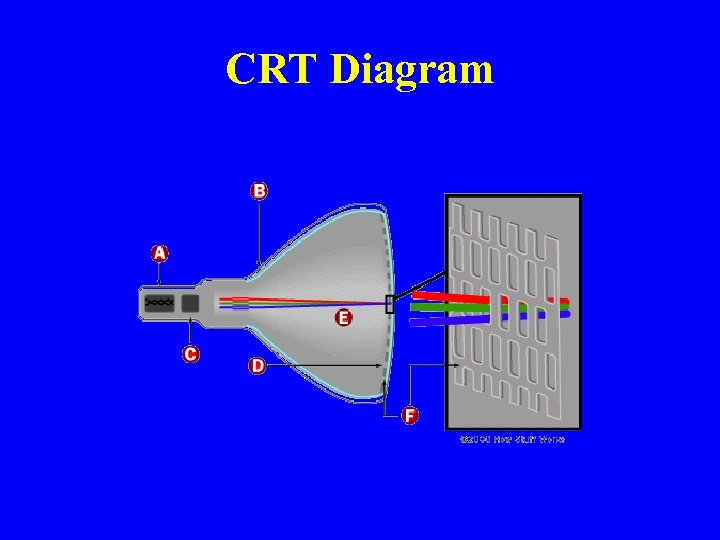CRT Diagram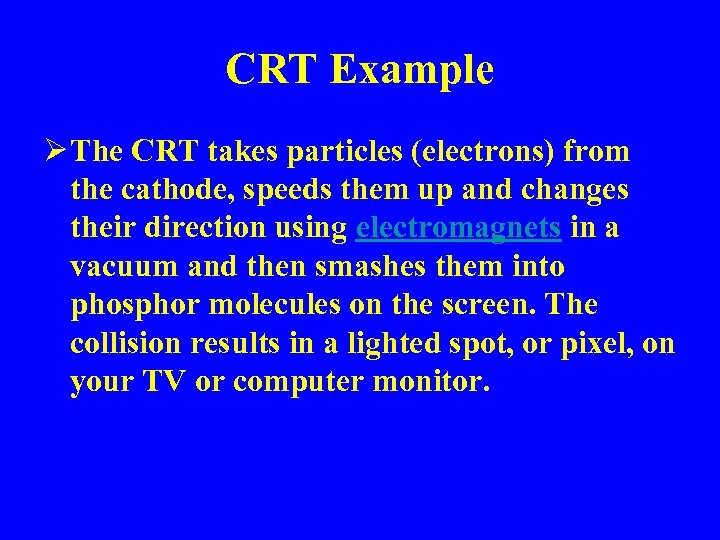CRT Example Ø The CRT takes particles (electrons) from the cathode, speeds them up and changes their direction using electromagnets in a vacuum and then smashes them into phosphor molecules on the screen. The collision results in a lighted spot, or pixel, on your TV or computer monitor.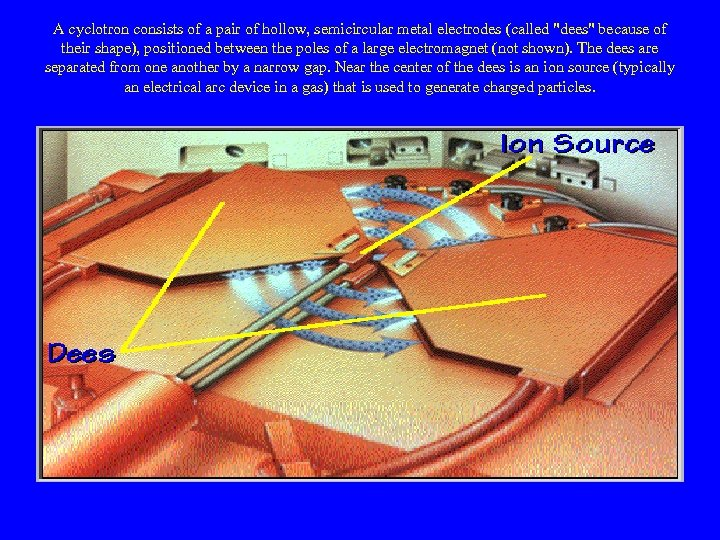A cyclotron consists of a pair of hollow, semicircular metal electrodes (called "dees" because of their shape), positioned between the poles of a large electromagnet (not shown). The dees are separated from one another by a narrow gap. Near the center of the dees is an ion source (typically an electrical arc device in a gas) that is used to generate charged particles.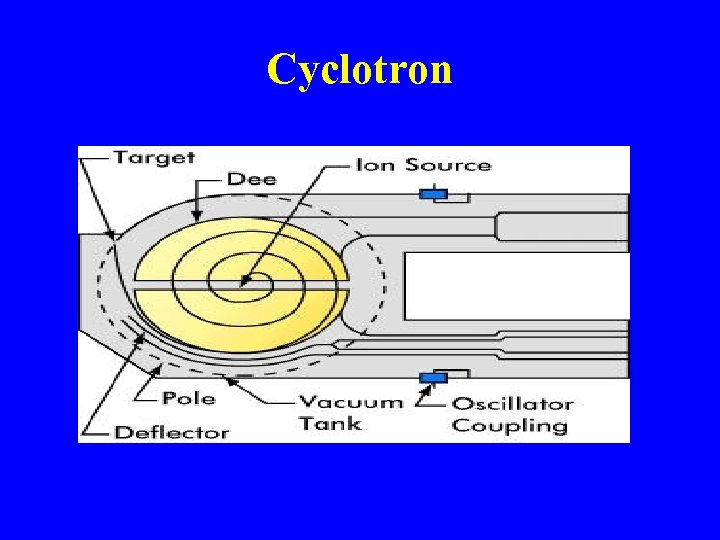Cyclotron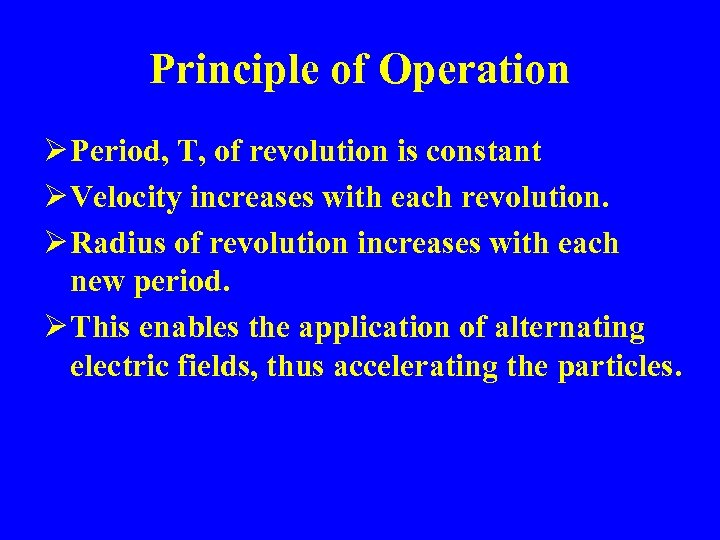Principle of Operation Ø Period, T, of revolution is constant Ø Velocity increases with each revolution. Ø Radius of revolution increases with each new period. Ø This enables the application of alternating electric fields, thus accelerating the particles.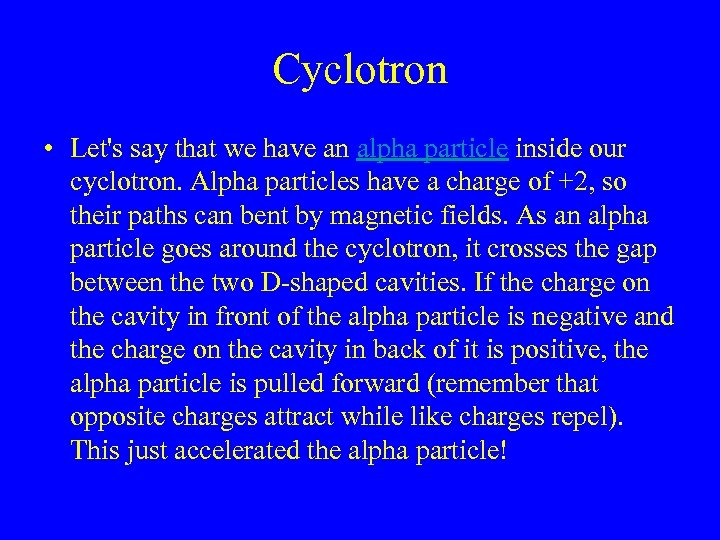Cyclotron • Let's say that we have an alpha particle inside our cyclotron. Alpha particles have a charge of +2, so their paths can bent by magnetic fields. As an alpha particle goes around the cyclotron, it crosses the gap between the two D-shaped cavities. If the charge on the cavity in front of the alpha particle is negative and the charge on the cavity in back of it is positive, the alpha particle is pulled forward (remember that opposite charges attract while like charges repel). This just accelerated the alpha particle!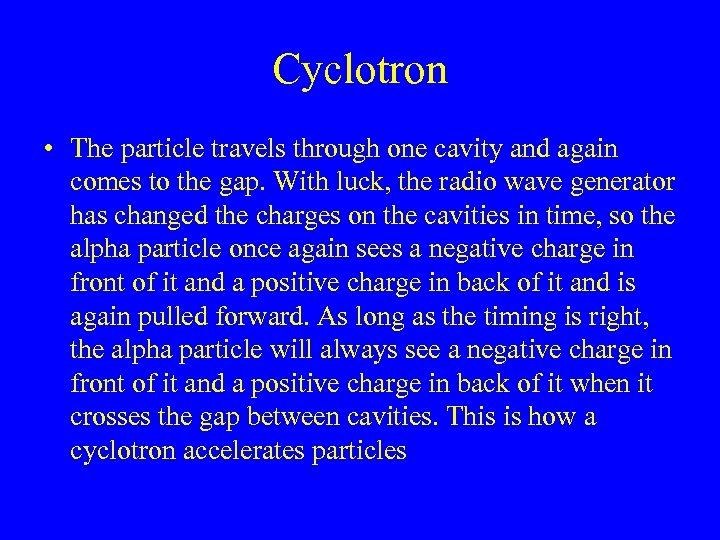Cyclotron • The particle travels through one cavity and again comes to the gap. With luck, the radio wave generator has changed the charges on the cavities in time, so the alpha particle once again sees a negative charge in front of it and a positive charge in back of it and is again pulled forward. As long as the timing is right, the alpha particle will always see a negative charge in front of it and a positive charge in back of it when it crosses the gap between cavities. This is how a cyclotron accelerates particles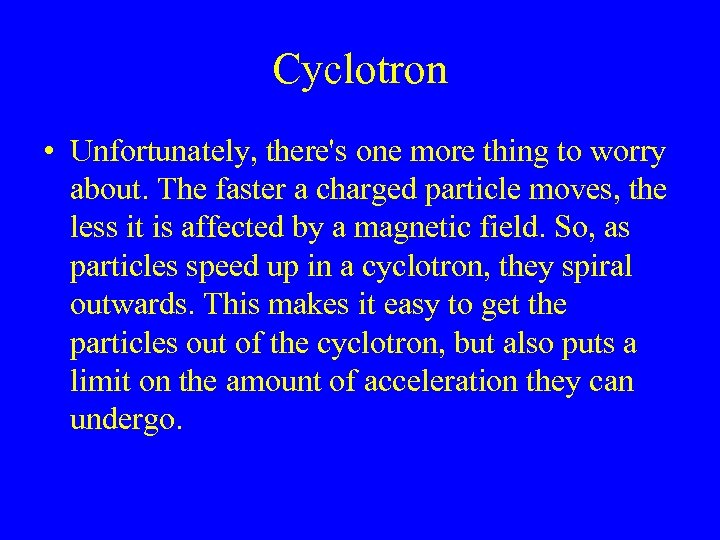Cyclotron • Unfortunately, there's one more thing to worry about. The faster a charged particle moves, the less it is affected by a magnetic field. So, as particles speed up in a cyclotron, they spiral outwards. This makes it easy to get the particles out of the cyclotron, but also puts a limit on the amount of acceleration they can undergo.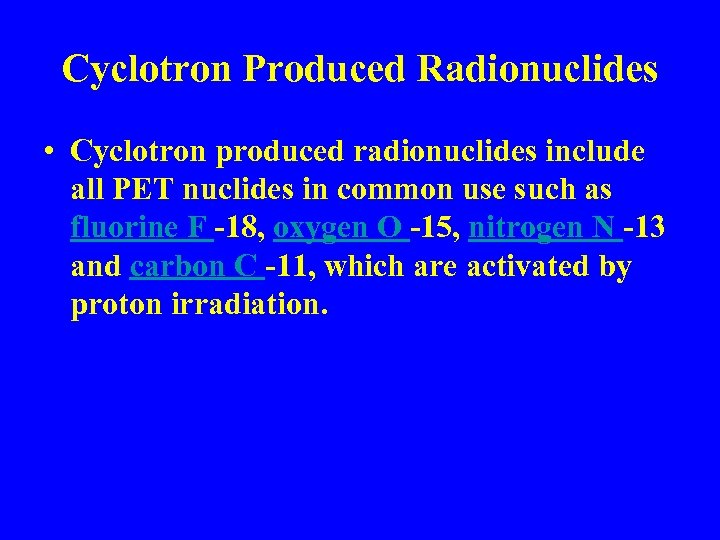Cyclotron Produced Radionuclides • Cyclotron produced radionuclides include all PET nuclides in common use such as fluorine F -18, oxygen O -15, nitrogen N -13 and carbon C -11, which are activated by proton irradiation.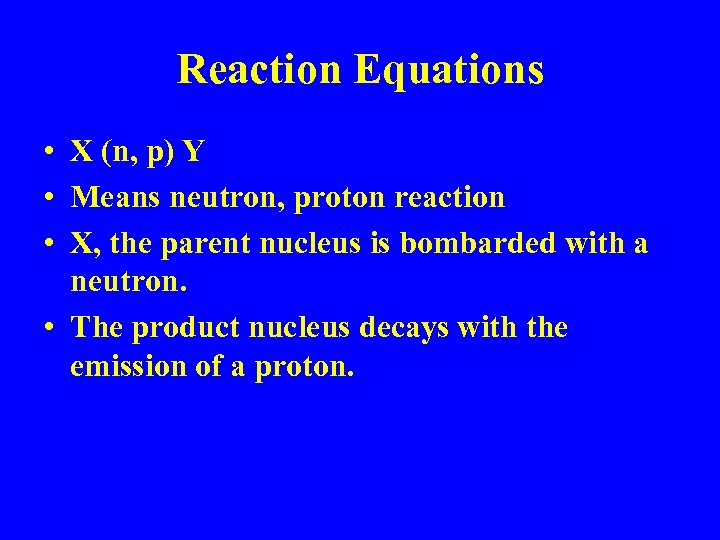Reaction Equations • X (n, p) Y • Means neutron, proton reaction • X, the parent nucleus is bombarded with a neutron. • The product nucleus decays with the emission of a proton.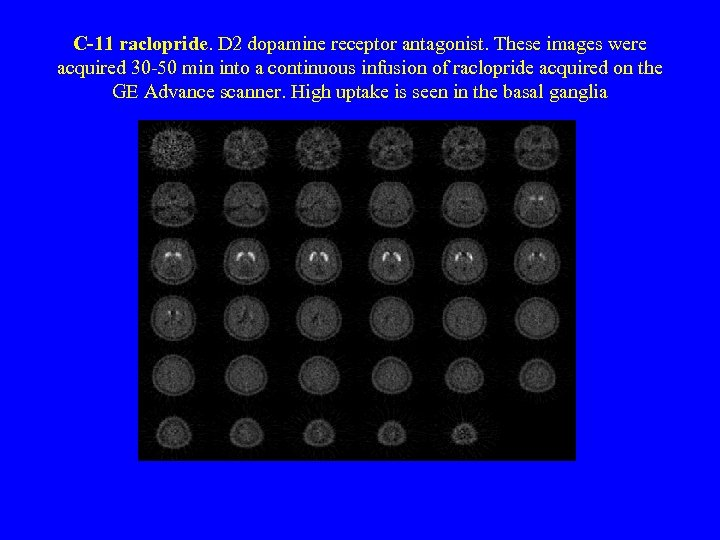C-11 raclopride. D 2 dopamine receptor antagonist. These images were acquired 30 -50 min into a continuous infusion of raclopride acquired on the GE Advance scanner. High uptake is seen in the basal ganglia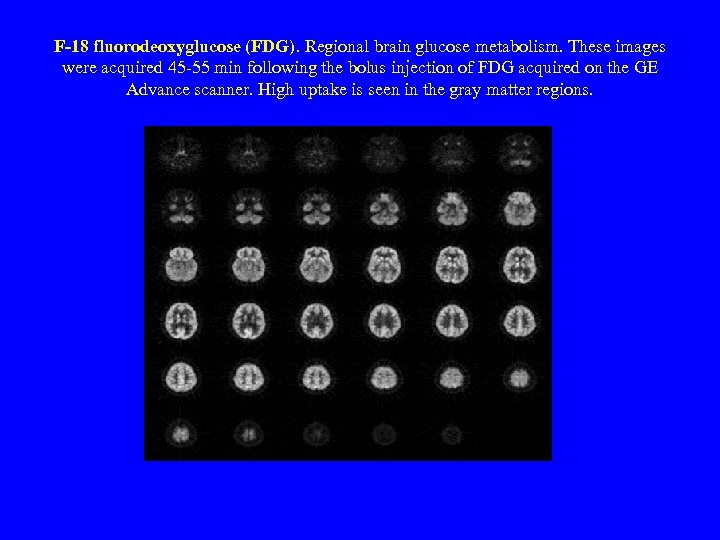F-18 fluorodeoxyglucose (FDG). Regional brain glucose metabolism. These images were acquired 45 -55 min following the bolus injection of FDG acquired on the GE Advance scanner. High uptake is seen in the gray matter regions.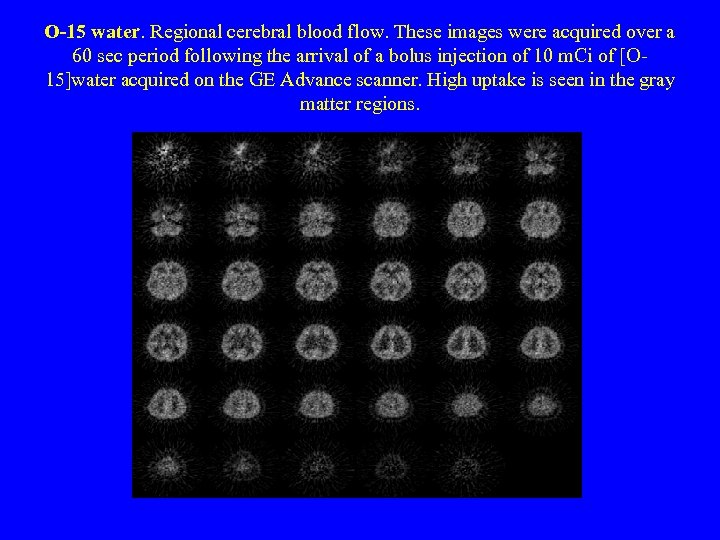O-15 water. Regional cerebral blood flow. These images were acquired over a 60 sec period following the arrival of a bolus injection of 10 m. Ci of [O 15]water acquired on the GE Advance scanner. High uptake is seen in the gray matter regions.